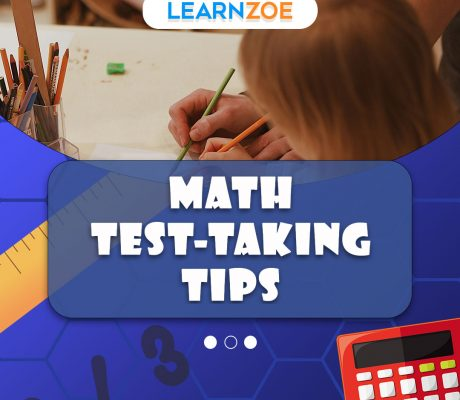# Category: Math Activities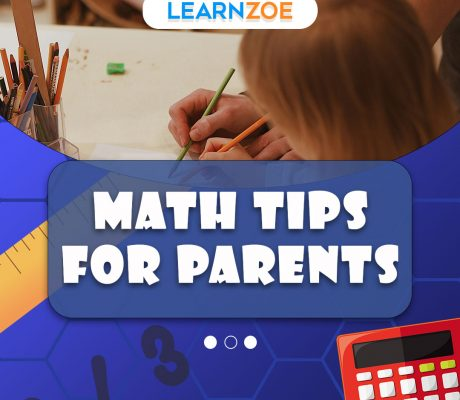### Math Tips for Parents

Math education in children’s early years plays a pivotal role in their cognitive development and future success. Why is math education important? Math education is critical because it forms the foundation for many skills that we use daily. It goes beyond numbers and calculations – it helps develop problem-solving abilities, logical thinking, and creativity. A study […]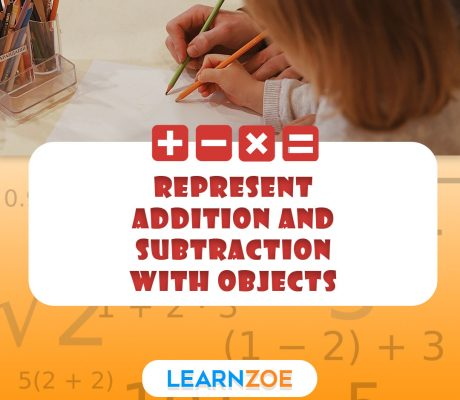### Represent Addition and Subtraction with Objects

Representing addition and subtraction with objects is a fundamental concept in mathematics education. It involves using physical objects, such as counters, blocks, or pictures, to model and visualize mathematical operations. This hands-on method helps students understand addition and subtraction better. They can see the items and move them to solve problems. Why representing math operations […]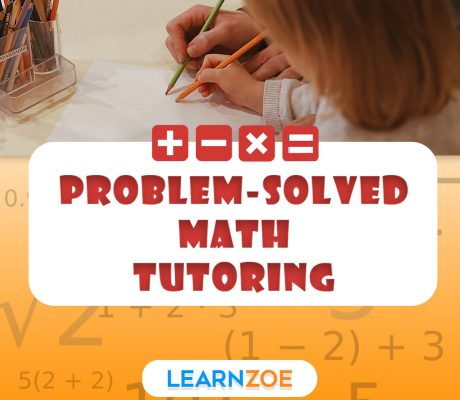### Problem-solved Math Tutoring

Math is integral to our daily lives in solving simple equations or handling complex problems. Choosing the best educational support, especially when it comes to math tutoring, can be a daunting task. In today’s section, we’ll dive into the importance and benefits of using problem-solved math tutoring to enhance learning. The Importance of Math Tutoring […]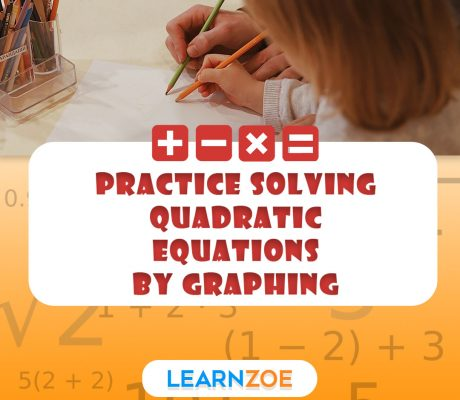### Practice Solving Quadratic Equations by Graphing

The study of quadratic equations forms a foundational part of algebra. A quadratic equation is a second-order polynomial equation in a single variable formed in the standard form ax + bx + c = 0. Here, ‘x’ represents an unknown variable, and ‘a, ‘b,’ and ‘c’ are constants. It is named quadratic, as quadratus is the […]